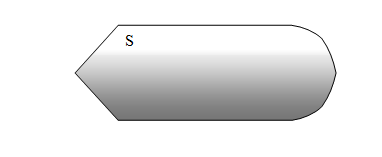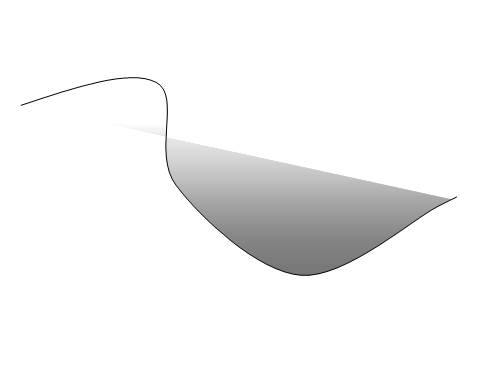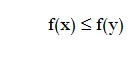# Non Linear Programming

### Basic Concepts and Principles

A non linear programming problem is a problem like
min f (x)
Subject to
gi (x) = 0

With
gi: Rn → R
f: Rn → R
x ∈ Rn
The problem is very different than linear case where through linear algebra methods could solve the problem. In the nonlinear case our work will be much more complex and profound, it evokes concepts as "continuity" or "differentiability" and covers various fields of analysis.

In the case of our problem as the objective function f, such as the constraints are differentiable and have continuous second partial derivativesthen issue may understand like a "special and extended" maximum or minimum value problem. Or a maximum or minimum restricted value problem.

### Extended Theory

Lets the feasible regionand lets the level sets of our functionThe feasible set of our problem is a set in the formor it could adopt an unbounded form likeand the objetive-function level setsIn general, the set constraints defines a region of R n .

We will make assumptions about this region (often topological) without paying much attention to the functions g i in themselves.

At first instance, we know that one Theorem that assures that every continuous function bounded in a compact (top and bottom).

This theorem together with the real numbers 13 axiom wich says that all real numbers set bounded above (below) has supperior (inferior) extreme.

This assures for us an existence of an optimum value for our problem in the bounded case.

Therefore, in the event that we have compactness in the constraints set we have an existence theorem and we can assure that there is a point and the feasible region S, such as for every x of S we have## Was useful? want add anything?

Post here

### Post from other users

Post here